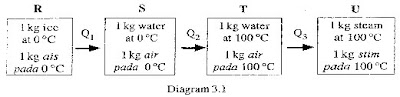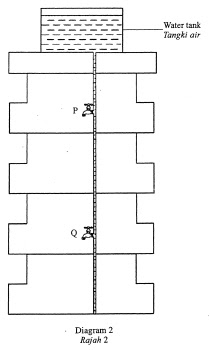## 15 October 2009

### Command Words

Command Words You Need To Know To Answer Questions Correctly

Name : Simply requires the technical name for a process, a chemical etc.Question:
Name the heat energy, Q1, which is absorbed from R to S.

Latent heat // Specific latent heat (of fusion)

State: State means you need to give only brief answers. This means that, detail explanations are not required. Only requires a simple statement, no reasoning is expected.

Question:
State one reason why the temperature at R and S is the same even though heat is supplied.

The heat needed to break the bonds between molecules // molecules move further apart // to change solid to liquid // to change physical form // kinetic energy doesn’t change.

Calculate: Used when a numerical answer is required. In general, working should be shown, especially where two or more steps are involved

Example:
Calculate the het energy, Q2, which is absorbed from S to T.
[The specific heat capacity of water, C=4200 J kg-10C-1]

Q = mcθ

1.0 x 4200 x 100
420 000 J // 4.2 x 105 J // 420 kJ

Meant: You need to remember only certain sentences used which are to conclude all aspects which are required.
Only a formal statement or equivalent paraphrase is required.

Questions:
What is meant by pressure?

Force per unit area // Force ÷ Area // F/A , where; F is force, A is area.

Compare: You need to state the difference or similarity about two tings.

Explain: Explain means that the details explanations are required to support a statement given. Important points/information need to be given specifically. General ideas are insufficient.Questions:
Based in Diagrams 2, compare the water pressure at P and Q. Explain your answer.

Pressure at Q is higher // Pressure at P is lower // Q > P // Q is higher // Q is more // Q is bigger // Q is more than P

Depth at Q is higher // Depth at P is lower // hQ > hP // HQ > HP > Q is deeper // Pressure increases as the depth increases

Complete:
Usually used where there are missing items in a table, diagram or words in a sentence paragraph.

Question: Complete the Table.
Distance from the concave mirror Characteristics of image
5 cm Virtual, upright and magnified
8 cm Virtual, upright and magnified
15 cm Real, inverted and magnified
200 cm Real, inverted and same size as the object.
25 cm Real, inverted and diminished

(b) Complete the ray diagram to show the image which is formed by the concave mirror. Mark the image with a letter I.(c) Complete the diagram (b) to show pattern of the waves after they pass through the gap
.Determine: Implies that the quantity concerned cannot be measured directly but is obtained by calculation, substituting measured or known values or other quantities into a standard formula.(a) Based on the graph in diagram (b),
(i) determine the focal length of the convex lens
f = 1/0.1
= 10 cm

(ii) determine the power of the convex lens.
P = 1/f
= 1/0.1
= 10 D

Suggest: Can be used in two main contexts, i.e. either to imply that there is no unique answer, or where this may not be formally ' in the syllabus', but the examiner thinks the candidate should be able to apply his knowledge to make a simple deduction.

Questions:The result obtained is shown by the graph in diagram above.
Suggest one way to determine the resistance of the bulb from the graph.

Describe: Requires the candidate to state in words (using diagrams where appropriate) the main points of the topic. It is often used with reference either to particular phenomena or to particular experiments.

Questions:
Describe how the current, I passing the heating element changes when the potential difference, V is increased from 0 to 240 V. Sketch a graph to support your description.

The current that flows through the heating element, increases as the potential difference across it increases.### SPM PRECAUTION

EXP: Measuring Instrument
- Avoid parallax error by placing the eye such that the line of view is perpendicular to the scale read. (for ammeter / voltmeter / measuring cylinder etc)
- Take into account zero error of instrument. (for caliper / micrometer / weight scale etc.)

Exp: Spring / Hookes Law
- Avoid parallax error by placing the eye such that the line of view is perpendicular to the scale of the ruler.

Exp: Heat
- Liquid must be stirred constantly so that temperature rises evenly.
- Alluminium block must be wrapped with insulating material to prevent heat lost.
- Thermometer bulb should be smeared with oil to give better thermal contact with the block.
- Avoid parallax error by placing the eye such that the line of view is perpendicular to the scale of the thermometer.

Exp: Light
- Experiment must be carried out in dark room.
- Lens, screen and object must be in line
- Avoid parallax error by placing the eye such that the line of view is perpendicular to the scale of the ruler.

Exp: Electricity
- All connections of wire & other device must be tight.
- Off the switch after readings are taken to prevent wires from heating up (resistance increase)
- Avoid parallax error by placing the eye such that the line of view is perpendicular to the scale of the ammeter/ voltmeter.

*NOTE
The below precautions are often written in exams:
- eyes must be perpendicular to the scale to avoid parallax error.
- repeat the experiment and find the average